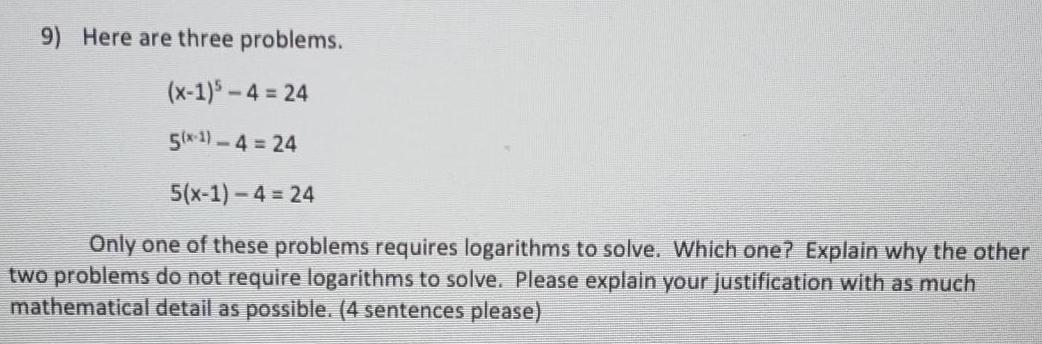Question:

# Here are three problems. (x-1)³-4 = 24 5^(x-1)-4 = 24

Last updated: 8/12/2022Here are three problems. (x-1)³-4 = 24 5^(x-1)-4 = 24 5^(x-1) — 4 = 24 Only one of these problems requires logarithms to solve. Which one? Explain why the other two problems do not require logarithms to solve. Please explain your justification with as much mathematical detail as possible.